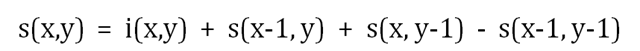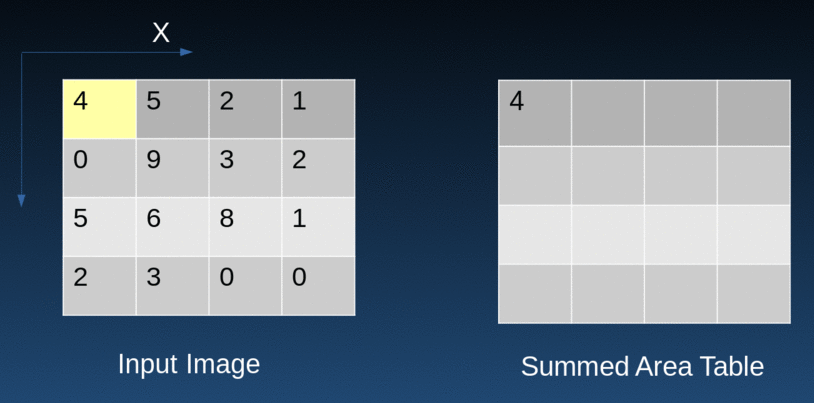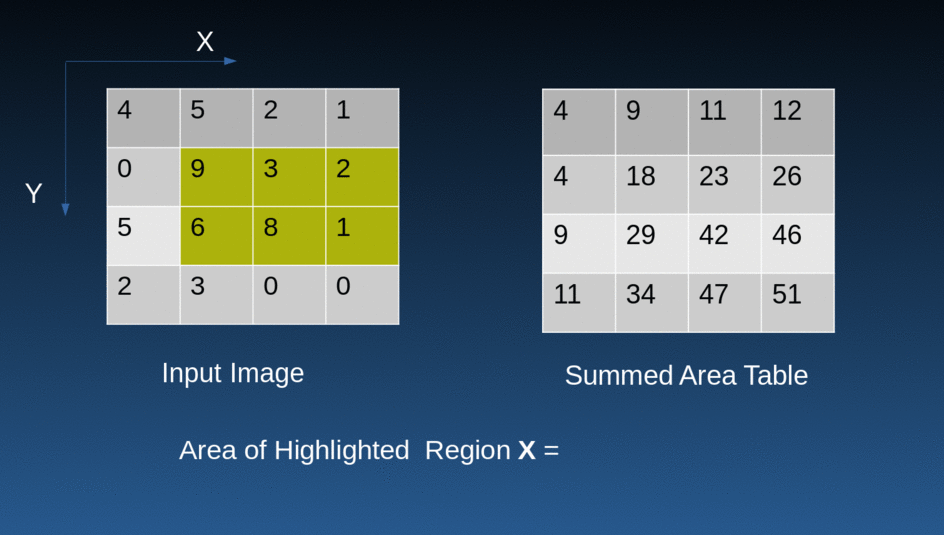# How to calculate Integral Image or Summed Area Table# Integral Image or Summed Area Table

An Integral image is where each pixel represents the cumulative sum of a corresponding input pixel with all pixels above and left of the input pixel. This algorithm enables rapid calculation of summations over image sub-regions. Any rectangular subset of such sub-region can be evaluated in constant time.

This concept was introduced by Viola & Jones and is also known as Summed Area Table. allow fast computation of rectangular image features since they enable the summation of image values over any rectangle image region in constant time i.e. computational complexity of O(1) instead of O(n).

An Integral Image is defined asEquation for calculating integral at pixel (x,y)

The SAT method has

• Space Complexity: O(M*N)

• Time Complexity for Range Sum Query: O(1)

• Time Complexity to Update a Value in Matrix: O(M*N)

• Efficiently computes the statistics like mean, standard deviation, etc in any rectangular window

Integral Image CalculationCalculation technique

Fast Area CalculationArea calculation for rectangular sub region of image

Sum = Bottom right + top left — top right — bottom left

### Uses

• region based statistical measures e.g. area sums, covariance, co-occurrence matrix

• Texture mapping

• detection of feature — HAAR

• adaptive threshold

• stereo correspondence

• The concept of integral images can be easily extended to continuous domain (using limits) and multidimensional images.

• O(1) Bilateral with Constant Spatial Filters

We at NAYAN have been using this quiet progressively to performa adaptive thresholding for License Plates from vehicles to better the output of OCR. So far we have been able to push our OCR accuracies from 91% to 97% by using a combination of techinques which also employ adaptive thresholding on unconstrained environments.

You can read more about how we implemetned smart parking finder here

References

1. http://apurvsaxena.blogspot.com/2012/06/integral-image.html

2. Speed-up Template Matching through Integral Image based Weak Classifiers by Wu.Tiriui et al. [Link]

3. Integral Images for Block Matching, Limare et. al. [Link]

4. https://datasciencechalktalk.com/2019/07/16/haar-cascade-integral-image

5. Constant Time O(1) Bilateral Filtering by Porikli Fatih [Link]

6. https://www.slideshare.net/egorodet/cpu-is-in-focus-again-implementing-dof-on-cpu

7. https://arxiv.org/pdf/1907.06154.pdf

p.s. Nayan is a platform that offers high precision services for traffic monitoring and road safety. Check out our website

Share

© 2019 NAYAN All Rights Reserved